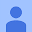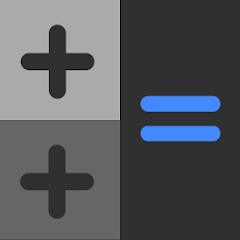# Calculator++

4.2
29 reviews
1K+Everyone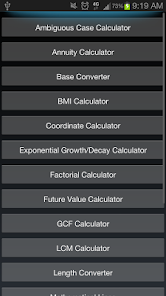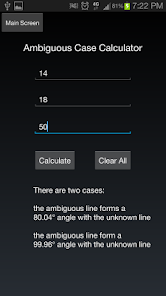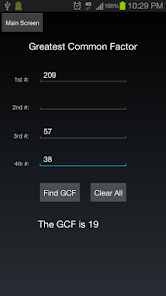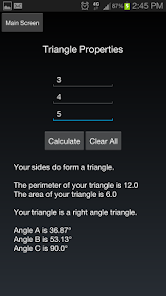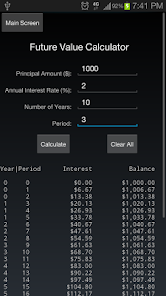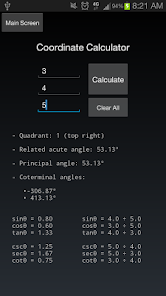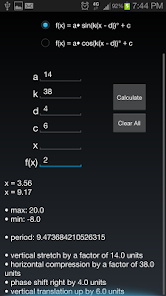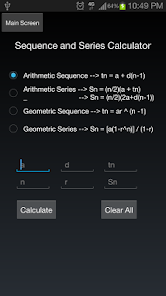Has 35 calculators that include (details at http://mikeyaworski.com/calculator++ ):

• Adding/Subtracting Fractions Calculator
• Ambiguous Case Calculator
• Annuity Calculator
• Base Converter
• Binary, Decimal and Hexadecimal Converter
• BMI (height in feet and inches, mass in pounds)
• Central Tendency Calculator (mean, median, mode, ordered set)
• Coordinate Calculator
• Cross and Dot Products Calculator
• Degree and Radian Converter
• e (4400 digits of e)
• Exponential Growth/Decay Calculator
• Factorial Calculator
• Fraction Reduction Calculator
• Future Value Calculator
• Greatest Common Factor (GCF; Greatest Common Divisor (GCD))
• Interest Calculator (Comound and Simple)
• Kinematics Variable Calculator (vi, vf, d, a, t)
• Lowest Common Multiple (LCM)
• Length Converter (to Metric)
• Mathematical Lines (slope, midpoint, length)
• Mark Calculator (weighted mean/average)
• Percentile Calculator
• Permutations/Combinations Calculator (P(n,r) and C(n,r) - n pick r; n choose r)
• PI (4400 digits of PI)
• Polynomial Roots Calculator (Quadratic, Cubic, Quartic)
• Prime Factorization Calculator
• Prime Number Finder (displays all factors as well)
• Prime Factorization Calculator
• Quadratic Roots Calculator (vertex of parabola too)
• Quartile Calculator
• Sequence and Series Calculator
• Sinusoidal Calculator
• Standard Deviation/Variance Calculator
• Triangle Properties (enter side lengths of any triangle; returns angles, area, perimeter, etc.)
• Z-Score Calculator

Please email michael@mikeyaworski.com if you have any calculator suggestions that I could add!
Updated on
May 27, 2017

## Data safety

Developers can show information here about how their app collects and uses your data. Learn more about data safetyNo information available
4.2
29 reviews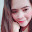Liezella Mantala
November 10, 2020
Good app.
Did you find this helpful?